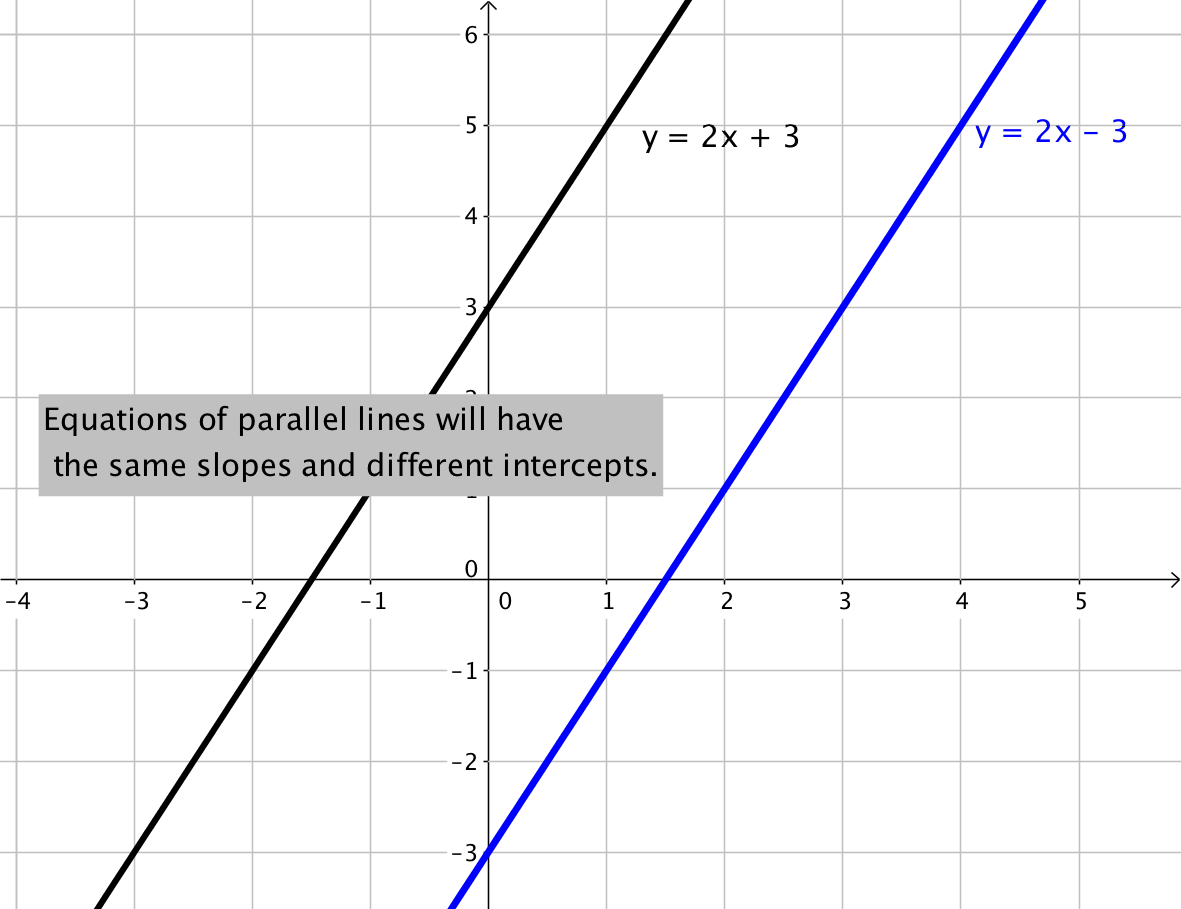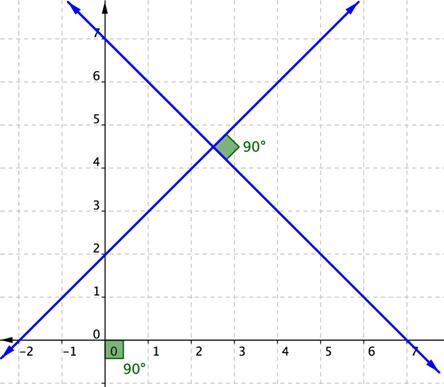## Slopes of Parallel and Perpendicular Lines

### Learning Outcomes

• Identify slopes of parallel and perpendicular lines

## Characterize the slopes of parallel and perpendicular lines

When you graph two or more linear equations in a coordinate plane, they generally cross at a point. However, when two lines in a coordinate plane never cross, they are called parallel lines. You will also look at the case where two lines in a coordinate plane cross at a right angle. These are called perpendicular lines. The slopes of the graphs in each of these cases have a special relationship to each other.

Parallel lines are two or more lines in a plane that never intersect. Examples of parallel lines are all around us, such as the opposite sides of a rectangular picture frame and the shelves of a bookcase.Perpendicular lines are two or more lines that intersect at a $90$-degree angle, like the two lines drawn on this graph. These $90$-degree angles are also known as right angles.Perpendicular lines are also everywhere, not just on graph paper but also in the world around us, from the crossing pattern of roads at an intersection to the colored lines of a plaid shirt.

### Parallel Lines

Two non-vertical lines in a plane are parallel if they have both:

• the same slope
• different $y$-intercepts

Any two vertical lines in a plane are parallel.

### Example

Find the slope of a line parallel to the line $y=−3x+4$.

### Example

Determine whether the lines $y=6x+5$ and $y=6x–1$ are parallel.

### Perpendicular Lines

Two non-vertical lines are perpendicular if the slope of one is the negative reciprocal of the slope of the other.

If the slope of the first equation is $4$, then the slope of the second equation will need to be $-\frac{1}{4}$ for the lines to be perpendicular.  You can also check the two slopes to see if the lines are perpendicular by multiplying the two slopes together. If they are perpendicular, the product of the slopes will be $−1$. For example, $4\cdot-\frac{1}{4}=\frac{4}{1}\cdot-\frac{1}{4}=-1$.

### Example

Find the slope of a line perpendicular to the line $y=2x–6$.

To find the slope of a perpendicular line, find the reciprocal, $\displaystyle \tfrac{1}{2}$, and then find the opposite of this reciprocal $\displaystyle -\tfrac{1}{2}$.

Note that the product $2\left(-\frac{1}{2}\right)=\frac{2}{1}\left(-\frac{1}{2}\right)=-1$, so this means the slopes are perpendicular.

In the case where one of the lines is vertical, the slope of that line is undefined and it is not possible to calculate the product with an undefined number. When one line is vertical, the line perpendicular to it will be horizontal, having a slope of zero ($m=0$).

### Example

Determine whether the lines $y=−8x+5$ and $\displaystyle y\,\text{=}\,\,\frac{1}{8}x-1$ are parallel, perpendicular, or neither.

Watch the video below for an example of how to determine when two lines are parallel or perpendicular.

## Contribute!

Did you have an idea for improving this content? We’d love your input.